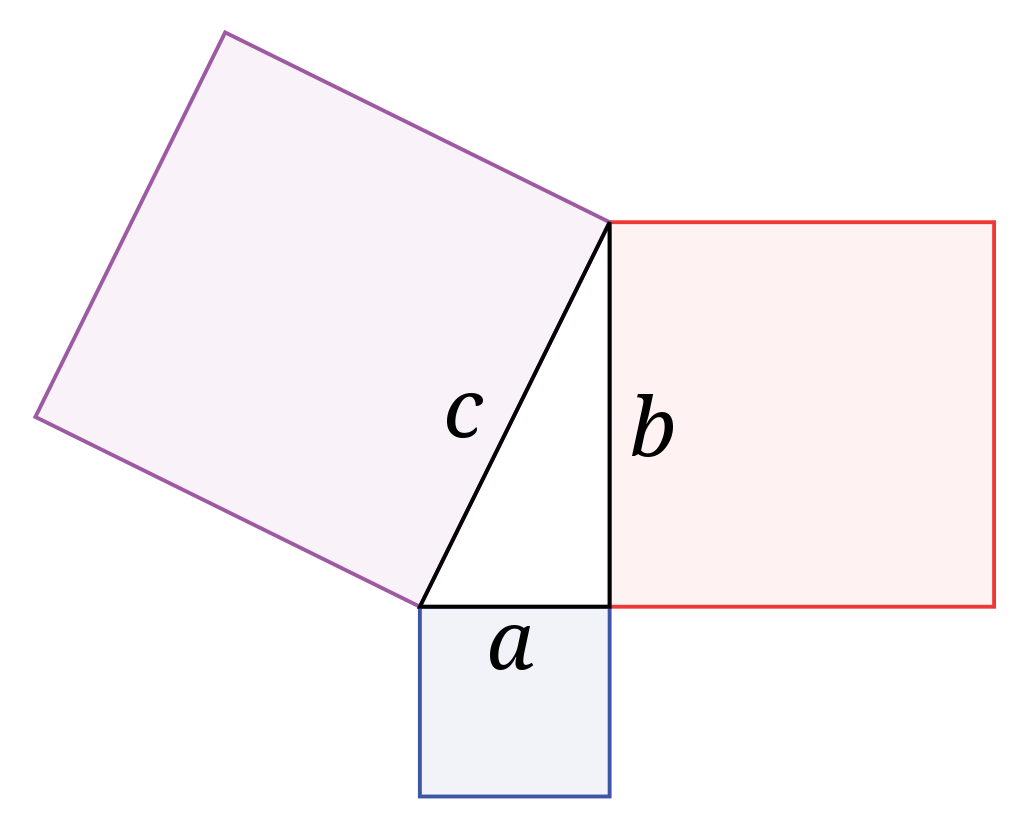Home Technology A Pythagoras’ Theorem Mess and its Experimental Hack

# A Pythagoras’ Theorem Mess and its Experimental Hack

346
0#### Remember the Pythagoras’ Theorem?

“A” squared plus “B” squared equals “C” squared. That is; Perpendicular squared plus Base squared equals to Hypotenuse squared. A theorem is often used to find the hypotenuse of a triangle.

#### In mathematics, a Pythagoras’ theorem, is a fundamental relation in Euclidean geometry, among the three sides of a right triangle.

A right triangle or right-angled triangle is a triangle in which one angle is a right angle (that is, a 90-degree). The relation between the sides and angles of a right triangle is the basis for trigonometry.

The largest side and the side opposite to the right angle is called the hypotenuse labeled as “c”. The sides adjacent to the right angle are called legs. Side “a” may be identified as Base and side “b” as Perpendicular.

This theorem can be expressed as an equation relating the lengths of the sides ab and c, often called the “Pythagoras’ equation”#### Pythagoras’ Equation.

It’s probably one of the few headaches that we faced during our Algebra class and our maths teacher spent so much time pushing it into your head.

The theorem has been proved using numerous methods. These methods are very diverse, including both geometric proofs and algebraic proofs. But, nstead of doing any maths, you can prove the theorem using water. All you need is a triangle, three square-shaped containers of the same thickness as that of a triangle and a circular axis to attach them. By filling the two sides of the triangle with water A and B then turning the axis over, the water in the two sides will exactly fill the container on the third side C.

Don’t believe? Check it out in a video below.